• 西南交通大学数学建模 Matlab绘图 Matlab 绘图 Matlab 绘图 手工作图 Matlab 绘图 Matlab 作图 Matlab 二维作图 plot 举例 Matlab 二维作图 图形的属性 点和线的基本属性 图形的基本属性 图形的其他属性 图形的其他...
• MATLAB二维绘图()操作绘图句柄显示特定的效果 预备知识：   在MATLAB中绘图用到的所有元素都是有唯一的句柄的，通过操作这个句柄可以修改这些元素，如绘图窗口figure的句柄是gcf，绘图中的axes的句柄是gca，这些...
MATLAB二维绘图(三)操作绘图句柄显示特定的效果
预备知识：
在MATLAB中绘图用到的所有元素都是有唯一的句柄的，通过操作这个句柄可以修改这些元素，如绘图窗口figure的句柄是gcf，绘图中的axes的句柄是gca，这些元素按照一定的等级分布，如axes的父级是figure等。如果需要修改这些元素的属性时，使用set函数，查看元素的属性时使用get函数。
示例：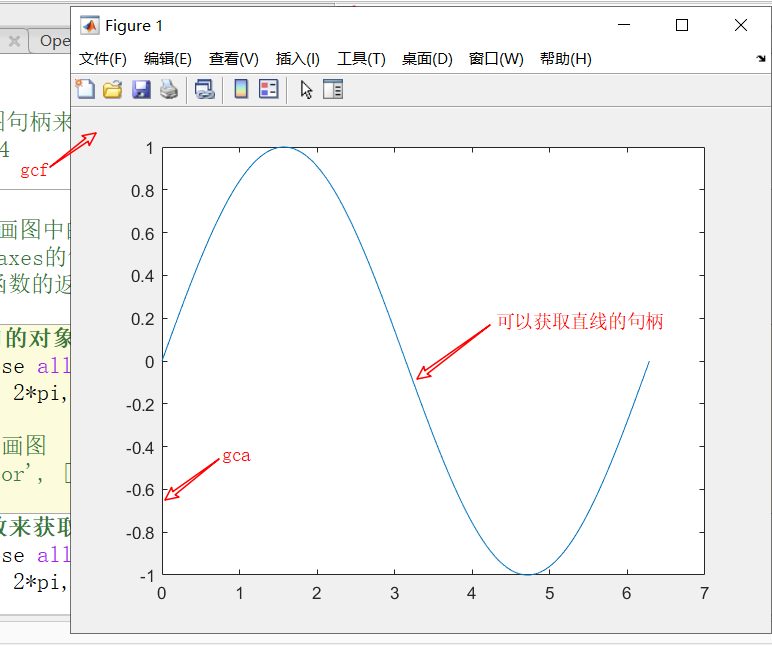1、通过set函数修改figure的背景颜色。示例：
%% 操作绘图窗口的对象句柄，来控制figure显示的颜色
clear; clc; close all;
x = linspace(0, 2*pi, 1000); % 获取均衡的点
y = sin(x);
plot(x,y);   % 画图
set(gcf, 'Color', [1 1 1]);  % 通过操作gcf句柄来控制figure的颜色显示

效果显示：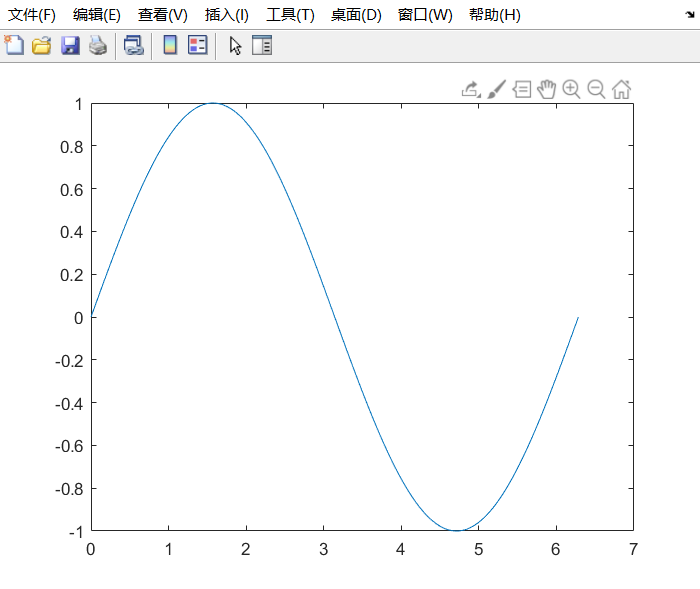使用get函数来查看gcf句柄的属性。通过查看这些属性可以进行相应的修改。示例：
%% 使用get函数来获取句柄的属性信息
clear; clc; close all;
x = linspace(0, 2*pi, 1000); % 获取均衡的点
y = sin(x);
plot(x,y);   % 画图
get(gcf)   % 在命令行窗口中输出属性信息

效果显示：(只是截取了一部分)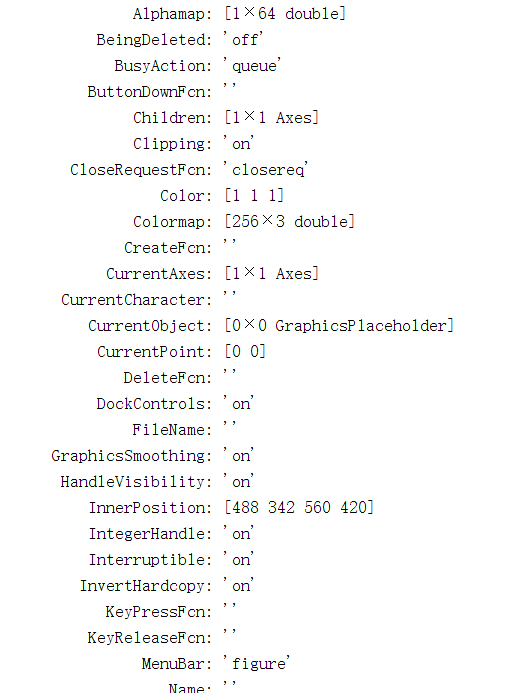说明：Children属性表示它的下一级元素，显示为axes。
2、通过plot函数获取直线的句柄来修改绘图直线的属性，示例：
%% 使用句柄h设置更加多样的曲线
clear; clc; close all;
x = linspace(0, 2*pi, 1000);
y = sin(x);
h = plot(x,y);  % 获取句柄h
set(h, 'LineStyle', '-.','LineWidth', 7.0, 'Color', 'g');  % 设置更加多样的曲线

效果显示：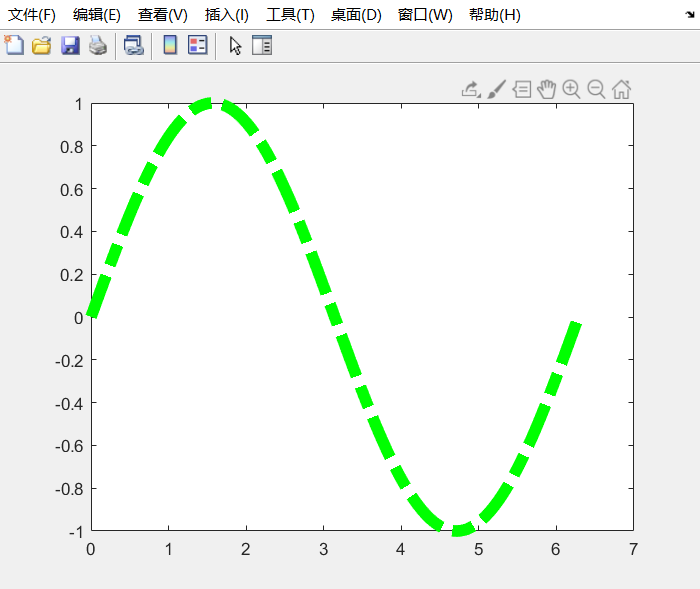说明：如果需要修改更多属性，使用get函数查看属性信息，并且修改相应的属性即可。
3、删除元素，使用delete函数可以删除绘图的元素。示例：
%% 使用delete删除曲线句柄h，则不会显示曲线
clear; clc; close all;
x = linspace(0, 2*pi, 1000);
y = sin(x);
h = plot(x,y);  % 获取句柄h
set(h, 'LineStyle', '-.','LineWidth', 7.0, 'Color', 'g');
delete(h);  % 如果删除了曲线对象，那么就不会有曲线显示了

效果显示：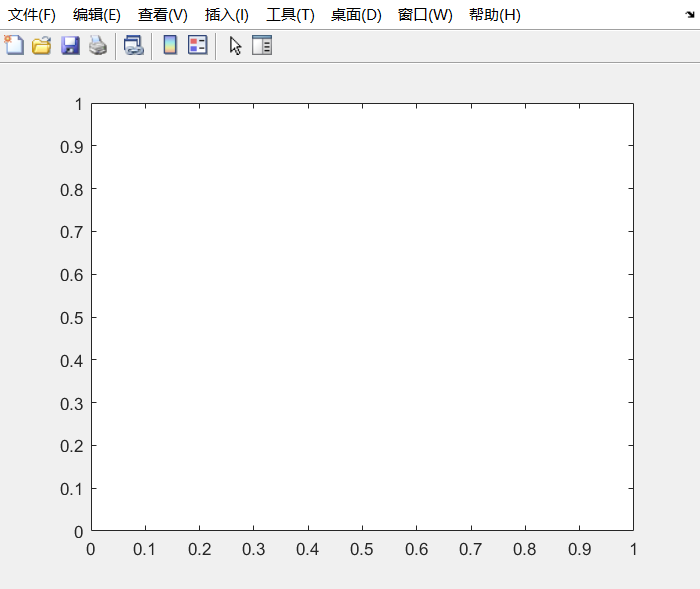操作元素句柄修改属性示例：
1、通过操作gca来设置axes坐标的范围。示例：
%% 设置曲线的父级axes
clear; clc; close all;
x = linspace(0, 2*pi, 1000);
y = sin(x);
h = plot(x,y);  % 获取句柄h
set(gca, 'XLim', [0, 2*pi]);  % 设置x的范围
set(gca, 'YLim', [-1.2, 1.2]);  % 设置y的范围

效果显示：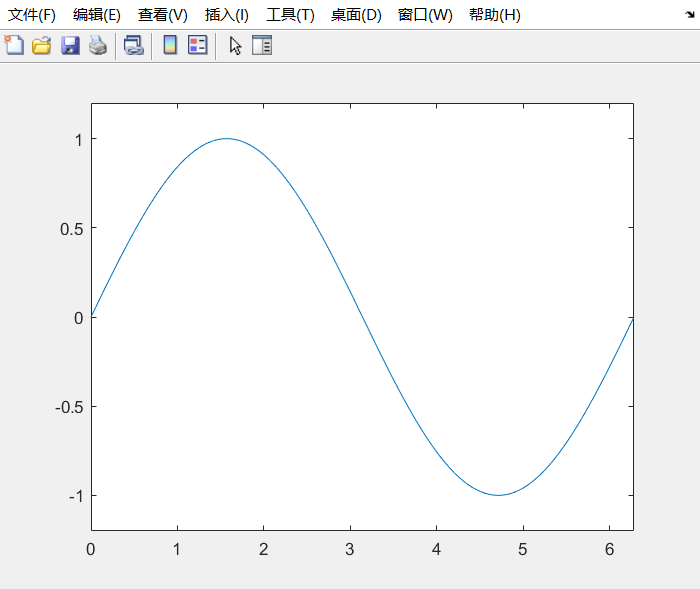2、设定axes控件的字体等信息。示例：
clear; clc; close all;
x = linspace(0, 2*pi, 1000);
y = sin(x);
h = plot(x,y);  % 获取句柄h
set(gca, 'FontName', 'console');  % 改变字体
set(gca, 'XTickLabel', {'0', 'p/2', 'p', '3p/2', '2p'});  % 可以显示特定的符号

效果显示：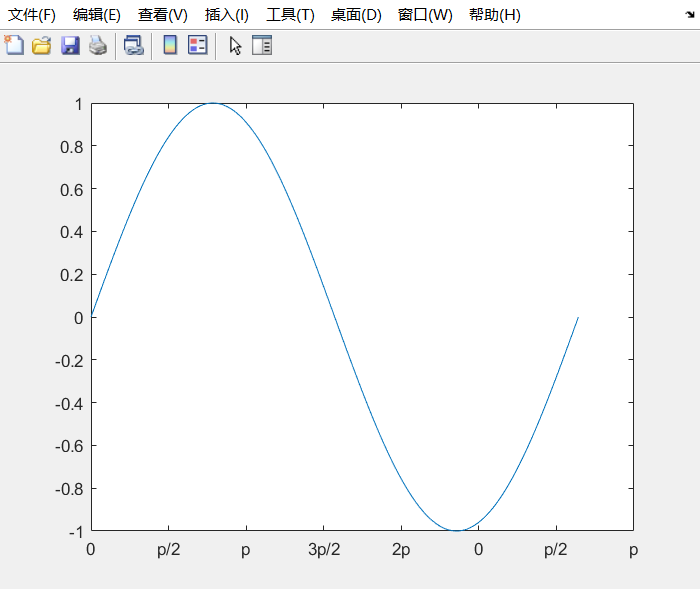想了解更多绘图信息，请看下面博文：
二维：
MATLAB二维绘图(一)使用plot函数进行简单绘图
MATLAB二维绘图(二)向图中添加标题，坐标轴，图标和文字信息
MATLAB二维绘图(四)其他特殊的二维绘图函数介绍及用法
fill填充函数解析及用法示例
三维：
MATLAB三维绘图(一)三维基础绘图
MATLAB三维绘图(二)使用隐函数作三维图
MATLAB三维绘图(三)绘制等值线图
MATLAB三维绘图(四)绘制特殊的三维图
MATLAB三维绘图(五)高级三维绘图
MATLAB三维绘图基础meshgrid函数的用法解析


展开全文• MATLAB绘图基础 1 MATLAB二维绘图 1.1 xy平面基本绘图命令 画多条曲线图 分割图形窗口 1.2 绘制图形的辅助操作 线型、标记符、颜色 线宽、标记点、字体等属性 图形设置 坐标轴设置 图形对象的修改 1.3 其他二维...
MATLAB绘图基础1 MATLAB的二维绘图1.1 xy平面基本绘图命令画多条曲线图分割图形窗口1.2 绘制图形的辅助操作线型、标记符、颜色线宽、标记点、字体等属性图形设置坐标轴设置图形对象的修改1.3 其他二维绘图函数
1 MATLAB的二维绘图
1.1 xy平面基本绘图命令

plot
x轴和y轴均为线性刻度

loglog
x轴和y轴均为对数刻度

semilogx
x轴为对数刻度，y轴为线性刻度

semilogy
x轴为线性刻度，y轴为对数刻度

figure
生成新的图形窗口

例子：
xd = [1, 5, 50];
yd = [30, 5, 5];
loglog(xd, yd, 'blacko-', 'MarkerFaceColor', [1, 0, 0]);
axis([10^0 10^2 10^0 10^2]);
grid on;axis equal

画多条曲线图
x=0:0.1:2*pi;
plot(x,sin(x),'ro',x,cos(x),'g*');分割图形窗口
调用格式为：subplot(n,m,k) 或 subplot(nmk)
n,m分别表示将窗口分割的行数和列数，k表示要画图部分的代号，表示第几个图形，nmk三个数可以连写,中间不用符号分开。
特殊情况：subplot(n,m,[a,b])
1.2 绘制图形的辅助操作
线型、标记符、颜色

线型
说明
标记符
说明
颜色
说明

-
实线（默认）
+
加号符
r
红色

- -
双划线
o
空心圆
g
绿色

：
虚线
*
星号
b
蓝色

-.
点划线
.
实心圆
c
青绿色

x
叉号符
m
洋红色

s
正方形
y
黄色

d
菱形
k
黑色

^
上三角形
w
白色

v
下三角形

<
左三角形

>
右三角形

p
五角星

h
六边形

线宽、标记点、字体等属性

LineWidth
线宽
数值，如0.5，1等，单位为points

MarkerEdgeColor
标记点边框线条颜色
颜色字符，如’g’, ’b’等

MarkerFaceColor
标记点内部区域填充颜色
颜色字符

MarkerSize
标记点大小
数值

FontName
字体名称

FontSize
字号大小

FontAngle
字体角度
‘normal’–正常；‘italic’–斜体；‘oblique’–倾斜;

FontWeight
字体的轻重
‘light’–减轻；‘normal’–正常；‘bold’–加粗

Box属性
决定图形坐标轴是否为方框形式
‘on’(有方框)；‘off’(无方框)

'ColorOrder’属性
设置多条曲线的颜色顺序
默认值为：[1 1 0;1 0 1;0 1 1;1 0 0;0 1 0;0 0 1]黄色 粉色 天蓝 红色 绿色 兰色，还有：[1 1 1]–白色；[0 0 0]–黑色

坐标轴方向属性
‘Xdir’，‘Ydir’，‘Zdir’
‘normal’–正常；‘reverse’–反向

坐标轴颜色属性
‘Xcolor’，‘Ycolor’，‘Zcolor’
颜色字符

坐标轴网格属性
‘Xgrid’，‘Ygrid’，‘Zgrid’
‘on’，‘off’

坐标轴的标尺属性
‘Xtick’，‘Ytick’，‘Ztick’
标度的位置,值为向量

‘Xticklabel’,‘Yticklabel’,‘Zticklabel’
轴上标度的符号,值与标度位置向量个数相同

图形设置
❥图形保持
hold on;

❥图形的网格
grid on %显示格线
grid off %关闭格线
grid %在两种状态之间切换

❥图形的图例
legend('y = sin(x)','y = cos(x)',……,pos);

pos指定图例框显示的位置：0–取最佳位置；1–右上角(缺省值)；2–左上角；3–左下角；4–右 下角；-1–图的右侧。
❥图形的说明文字
A.text函数：按指定位置在坐标系中写出说明文字
text(x1,y1,'字符串','选项');

选项’决定x1,y1的坐标单位，如没有选项，则x1,y1的坐标单位和图中一致。
B.gtext函数：按照鼠标点按位置写出说明文字
gtext('字符串');

坐标轴设置
❥坐标轴的控制
axis off %隐去坐标轴
axis on %显示坐标轴

❥坐标轴的说明
xlabel('Input Value'); %x轴注解
ylabel('Function Value'); %y轴注解

❥坐标轴的标题
title('Two Trigonometric Functions'); %标题

❥坐标轴刻度设置
set(gca,’XTick’,[0 1 2]);  %X坐标轴刻度数据点位置
set(gca,'XTickLabel',{'a','b','c'});  %X坐标轴刻度处显示的字符

❥坐标轴范围设置
axis([xmin xmax ymin ymax]); %设置坐标轴在指定的区间
axis auto %使用默认设置
axis manual %冻结当前坐标轴范围，以后叠加绘图都在当前坐标轴范围内显示
axis tight %采用紧密模式设置当前坐标轴范围

❥坐标轴比例设置
axis equal %等比例坐标轴
axis square %将坐标轴区域调整为正方形
axis normal %自动调整纵横轴比例，使当前坐标轴范围内的图形显示达到最佳效果

范围选项和比例设置可以联合使用，默认的设置为axis auto normal
❥坐标轴边框设置
box on; %显示边框
box off; %关闭边框
box; %切换状态

❥坐标轴位置设置
x轴位置：
set(gca,'XAxisLocation','bottom'); %将x轴的位置设置在底部(默认)。
set(gca,'XAxisLocation','top'); %将x轴的位置设置在顶部。
set(gca,'XAxisLocation','origin'); %将x轴的位置设置在y=0处。

y轴位置：
set(gca,'YAxisLocation','left'); %将y轴的位置设置在左边(默认)。
set(gca,'YAxisLocation','right'); %将y轴的位置设置在右边。
set(gca,'YAxisLocation','origin'); %将y轴的位置设置在x=0处。

❥坐标轴方向设置
x轴方向：
set(gca,'XDir','normal'); %将x轴方向设置为普通(从左到右递增)。
set(gca,'XDir','reverse'); %将x轴方向设置为反向(从右到左递增)。

y轴方向：
set(gca,'YDir','normal'); %将y轴方向设置为普通(从下到上递增)。
set(gca,'YDir','reverse'); %将x轴方向设置为反向(从上到下递增)。

图形对象的修改
❥set函数：用于设置句柄所指的图形对象的属性。
格式为：set(句柄, 属性名1, 属性值1, 属性名2, 属性值2, ……)
例子：
h=plot(x,y);
set(h,'Color', [1,0,0]); %将句柄所指曲线的颜色设为红色

❥get函数：获取指定句柄的图形对象指定属性的当前值。
格式为：get(句柄,‘属性名’)
执行后可返回X轴的当前颜色属性值[0,0,0](黑色）。
例子：
get(gca,'Xcolor'); %获得X轴的当前颜色属性值

❥如果没有设置句柄，则可以使用下列函数获得。
gcf：获得当前图形窗口的句柄；
gca：获得当前坐标轴对象的句柄；
gco：获得当前对象的句柄。
例子：
t=-pi:pi/20:pi;
y=sin(t);
plot(t,y);
set(gca,'xtick',[-pi:pi/2:pi],'xticklabel',['-pi','-pi/2','0','pi/2','pi']); %设置坐标轴的显示刻度
set(gcf, 'Color', [1,1,1]); %将图形窗口底色设为白色
set(gca, 'Xcolor', [0,1,0]); %X轴的颜色设为绿色

例子：设置坐标轴字体名称，大小，角度，轻重的同时，也会设置坐标轴注解的字体；之后可以单独设置Y轴注解的字体名称，大小。
set(gca,'FontName','Times New Roman','FontSize',14,'FontWeight','bold','FontAngle','italic')
ylabel('前角(°)','FontName','宋体','FontSize',10.508)

对字体的设置也可以用在title，xlabel，ylabel等中。
例子：
a=linspace(1,2,10);
plot(a,'--pr','linewidth',1.5,'MarkerEdgeColor','r','MarkerFaceColor','m','MarkerSize',10);
legend('a','Location','best');
title('a','FontName','Times New Roman','FontWeight','Bold','FontSize',16);
xlabel('T','FontName','Times New Roman','FontSize',14);
ylabel('a','FontName','Times New Roman','FontSize',14,'Rotation',0);
axis auto equal
set(gca,'FontName','Times New Roman','FontSize',14);

1.3 其他二维绘图函数

函数
说明

bar
长条图

errorbar
图形加上误差范围

fplot
较精确的函数图形

polar
极座标图

hist
累计图

rose
极座标累计图

stairs
阶梯图

stem
针状图

fill
实心图

feather
羽毛图

compass
罗盘图

quiver
向量场图

pie
饼图

❥长条图bar

bar
绘制二维竖直直方图

bar3
绘制三维竖直直方图

barh
绘制二维水平直方图

bar3h
绘制三维水平直方图

调用格式为：
bar(x,y); 或 bar(y); %默认条形类型为分组
bar(x,y,width); 或 bar(y,width); %指定每个直方条的宽度，如width>1，则直方条会重叠，默认值为width=0.8
bar(…,'grouped'); %使同一组直方条紧紧靠在一起
bar(…,'stack'); %把同一组数据描述在一个直方条上

例子：
x=1:10;
y=rand(size(x));
subplot(221);bar(x,y);%默认条形类型为分组
y=[9 8 6;2 5 8;6 2 9;5 8 7;9 4 2];
subplot(222);bar(y);%竖直直方图
subplot(223);barh(y);%水平直方图
subplot(224);bar(y,'stack');%'stack'制定条形类型为叠加
colormap(cool);%将当前颜色映象色调改为cool❥图形加上误差范围errorbar
调用格式为：
errorbar(B,X); %X为误差
errorbar(A,B,X); %X为误差
errorbar(A,B,X,Y); %X为负向误差，Y为正向误差

例子：
T=1:1:6;  %月份
A=[12,11,7,7,6,5];  %A地各月的平均值
V=[0.5,0.4,0.3,1,0.3,0.5];  %A地各月的方差值
A2=[10,8,5,4,3,3];  %B地各月的平均值
V2=[0.4,0.3,0.4,0.6,0.3,0.5];    %B地各月的方差值
subplot(121);errorbar(T,A,V,'r-o');
hold on;errorbar(T,A2,V2,'b-s');  %注意'-o'中的‘-’去掉后画出来的图是各个孤立的点
xlabel('月份');ylabel('降雨量/cm');
Vp=[0.3,0.6,0.5,0.5,0.3,0.2]; %A地各月的正向方差
Vn=[0.5,0.5,0.3,1,0.5,0.4];   %A地各月的负向方差
subplot(122);errorbar(T,A,Vn,Vp,'linewidth',2);
xlabel('月份');ylabel('降雨量/cm');❥较精确的函数图形fplot
函数特点：
绘图的数据点是自适应产生的。在函数平坦处,所取数据点比较稀疏;在函数变化剧烈处,将自动取较密的数据点。

调用格式为：
fplot(fun,limits,tol,linespec);
fun:函数名,可以是MATLAB已有函数、自定义的M函数或字符串定义的函数。
limits:表示绘制图形的坐标轴取值范围,有两种方式:[xminxmax]和[xminxmaxyminymax]。
tol:相对误差,默认值为2e-3。
linespec:表示图形的线型、颜色和数据点等设置。

例子：
x=-1:0.1:1; %定义域取值范围和步长
fplot('sin(1./x)',[0.02 0.2]); %[0.02 0.2]是绘图范围❥极座标图polar
调用格式为：
polar（theta，rho，选项）; %theta为极坐标极角，rho为极径，选项的内容和plot函数相似

例子：
theta=0:0.01:2*pi;
r=cos(4*theta);
polar(theta,r,'b');❥累计图hist
对于大量的资料，我们可用hist来显示资料的分情况和统计特性。
调用格式为：
hist(x); %x是一维向量，函数的作用是将x中的最小和最大值之间的区间等分成10等份，输出的直方图横坐标是x值，纵坐标是该值的个数
[a,b]=hist(x,n); %其中x是一维向量，函数功能是将x中的最小和最大值之间的区间等分n份，横坐标是x值，纵坐标是该值的个数。返回的a是落在该区间内的个数，b是该区间的中心线位置坐标。

例子：
x=[1,2,3,4,5,6,1,2,5,4,7,8,5,6,4,6];
subplot(121),hist(x);
x=randn(200, 1); %产生5000个m=0,s=1的高斯乱数
subplot(122),hist(x,15); %20代表长条的个数❥极座标累计图rose
rose和hist很接近，只不过是将资料大小视为角度，资料个数视为距离，并用极座标绘制。
例子：
x=randn(1000, 1);
rose(x);❥阶梯图stairs
调用格式为：
stairs(Y);
stairs(X,Y);

例子：
x=linspace(-2*pi,2*pi,40);
subplot(211),stairs(x,sin(x))
hold on;plot(x,sin(x),':*');
rng('default');
n=rand(1,10);
subplot(212),stairs(n);❥针状图stem

stem(X,Y)
绘制二维针状图

stem3(X,Y,Z)
绘制三维针状图

调用格式为：
stem(Y); %将数据序列Y从x轴到数据值按照茎状形式画出，以圆圈终止。如果Y是一个矩阵，则将其每一列按照分隔方式画出。
stem(X,Y); %在X的指定点处画出数据序列Y
stem(...,'filled'); %以实心的方式画出茎秆
stem(...,'LINESPEC'); % 按指定的线型画出茎秆及其标记

例子：
x=0:0.2:10;
y=sin(x).*exp(-x/3);
subplot(221),stem(x,y); %绘制二维针状图
subplot(222),stem(x,y,':sr'); %改变线型、标记点形状和颜色
subplot(223),stem(x,y,'fill');%填充颜色
A=[1,2,3;4,6,7];
subplot(224),stem3(A); %绘制三维针状图❥实心图fill

fill
绘制二维填充图

fill3
绘制三维填充图

调用格式为：
fill(X,Y,C); %创建填充多边形顶点的颜色从C中指定的X和Y的数据是一个向量或矩阵用于作为颜色表索引。如果C是一个行向量，长度（C）必须大小相等的（x，2）和大小（Y，2）；如果C是一个列向量，长度（C）必须大小相等的（x，1）和大小（Y，1）。如果有必要，填充闭合多边形的顶点连接最后一。
fill(X,Y,ColorSpec); %二维多边形填充指定的X和Y与指定的colorspec颜色
fill(X1,Y1,C1,X2,Y2,C2,...); %指定多个二维填充区域
fill(...,'PropertyName',PropertyValue); %允许您指定一个补丁的图形对象的属性的名称和值。
h=fill(...); %返回一个向量处理图形对象的补丁，每个补丁的对象一柄
fill3(X,Y,Z,C)
fill3(X,Y,Z,ColorSpec)
fill3(X1,Y1,Z1,C1,X2,Y2,Z2,C2,...)
fill3(...,'PropertyName',PropertyValue)
fill3(ax,...)
h=fill3(...)

例子：
x=-2*pi:pi/100:2*pi;
y=sin(x);
subplot(121),fill(x,y,'g');%绘制二维填充图
axis([-7,7,-1.5,1.5])
hold on;
fill([0:pi/100:pi],sin([0:pi/100:pi]),'r');
fill([-2*pi:pi/100:-pi],sin([-2*pi:pi/100:-pi]),'r');
hold off
X=[0;1;1;0];
Y=[1;1;0;0];
Z=[1;1;0;0];
subplot(122),fill3(X,Y,Z,[0.1 0.2 0.3]);%绘制三维填充图❥羽毛图feather
调用格式为：
feather(x,y) 函数绘制由向量参量x与y构成的速度向量，沿水平轴方向，从均匀间隔点以箭头发射出来；
feather(z) 函数绘制羽毛图。参量z是一个复数，则feather(z)相当于compass(real(z)，imag(z))；
feather(...,LineSpec) 函数绘制羽毛图。参量LineSpec指定的线型、标记符号、颜色等属性画出羽毛图；
H = feather(...) 函数返回羽毛图中线型对象的句柄H。

例子：
theta=linspace(0, 2*pi, 20);
z = cos(theta)+i*sin(theta);
subplot(211),feather(z);
theta=-pi/2:pi/16:pi/2;
r=2*ones(size(theta));
[u,v]=pol2cart(theta,r);%把极坐标（柱坐标）转换为笛卡尔坐标（指平面直角坐标或空间直角坐标）。
subplot(212),feather(u,v);❥罗盘图compass
调用格式为：
compass(x,y); %函数绘制一个由原点出发由(x,y)组成的向量箭头图形；
compass(z); %等价于compass（real(z),imag(z))；
compass(...,LineSpec); %函数用参量LineSpec指定箭头的线型、标记符号、颜色等属性；
h=compass(...); %函数返回line对象的句柄给h。

例子：
theta=linspace(0,2*pi,20);
z=cos(theta)+i*sin(theta);
subplot(121),compass(z,'-.r');
x=1:100;
y=rand(1,100);
subplot(122),compass(x,y);❥向量场图quiver
调用格式为：
quiver(x,y,u,v); %用来绘制二维方向箭头，表示通过在(x, y)指定的位置绘制小箭头来表示以该点为起点的向量(u,v)。x,y,u,v必须是相同阶数。
quiver(u,v); %在x-y面上绘制向量(u,v)，由于没有指定向量的起点，所以，matlab将在x-y面上均匀的取若干个点作为起点。

例子：
u=[1 1 1; 1 1 1];v=u;
subplot(121),quiver(u, v);
x=[0 0 0 0];y=x;%指定起点为(0,0)
u=[1 0 -1 0];v=[0 1 0 -1];
subplot(122),quiver(x, y, u, v);❥饼图pie

pie(X)
绘制二维饼图

pie3(X)
绘制三维饼图

调用格式为：
pie(X); %利用向量X中的数据描绘饼图，X中的数据被看做频数，饼图中比率的获得：X中的元素x[i]/sum(X)。当X中所有元素的和sum（X）< 1.0时，图形不是一个整圆。
pie(X,{'...', '...', ...}); %‘...’是标注。
pie(X,y); %将一些扇区从饼图中分离出来，y为一个与X尺寸相同的矩阵，其非零元素所对应X矩阵中的元素从饼图中分离出来。

例子：
x=[5.5 74.7 44.5 33.2 46.6];
subplot(231),pie(x); %不分离饼图
subplot(232),pie(x,{'1', '2', '3','4','5'}); %‘...’是标注。
subplot(233),pie(x,[0 0 0 0 1]);%分离饼图，将第五个元素分离出来
x1=[0.2 0.3 0.4];
subplot(234),pie(x1); %不完整饼图
x2=[1 2 4 3 5];
subplot(235),pie3(x2); %绘制三维饼图
subplot(236),pie3(x2,[0 1 0 1 0]); %将第二、四个元素分离出来展开全文• MATLAB三维绘图(一)三维基础绘图   三维图就是在维图的基础上多增加了一维，实质上在MATLAB中绘制的都是三维图。简单看一下维图是怎样变成三维图的，使用plot3函数可以很清楚的看出来。示例： %% 简单理解三维...
MATLAB三维绘图(一)三维基础绘图
三维图就是在二维图的基础上多增加了一维，实质上在MATLAB中绘制的都是三维图。简单看一下二维图是怎样变成三维图的，使用plot3函数可以很清楚的看出来。示例：
%% 简单理解三维画图
clear; clc; close all;
x=0:0.1:5*pi;
z1=sin(x);
z2=sin(2.*x);
z3=sin(3.*x);
y1=zeros(size(x));
y3=ones(size(x));
y2=y3./2;
plot3(x,y1,z1,'r',x,y2,z2,'b',x,y3,z3,'g');  % 画出三条不同的直线显示的是三维图像
grid on; % 显示网格
xlabel('x-axis'); ylabel('y-axis'); zlabel('z-axis');

效果显示：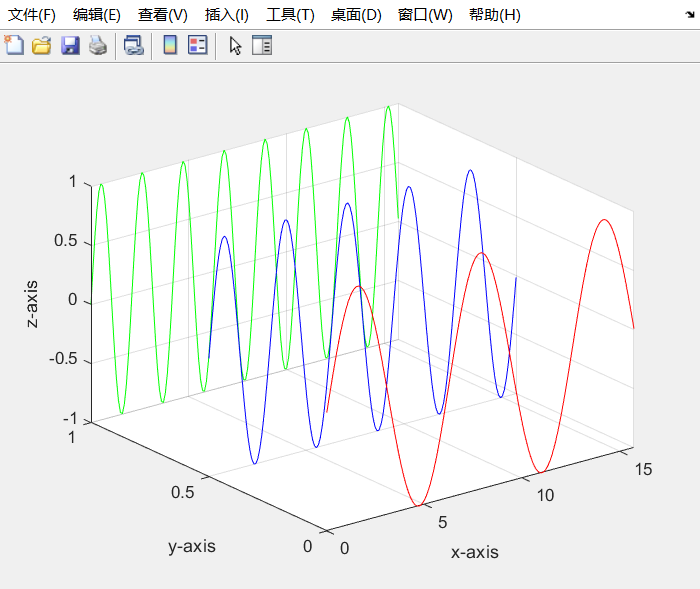1、使用最简单的三维画图函数plot3来认识一下三维图。示例1：弹簧图
%% 螺旋状图(弹簧)
clear; clc; close all;
t = 0:pi/50:10*pi;
plot3(sin(t),cos(t),t);  % 使用plot3进行绘制三维曲线图
grid on;   % 显示网格
axis square;   % 坐标边框尺度大小相同

效果显示：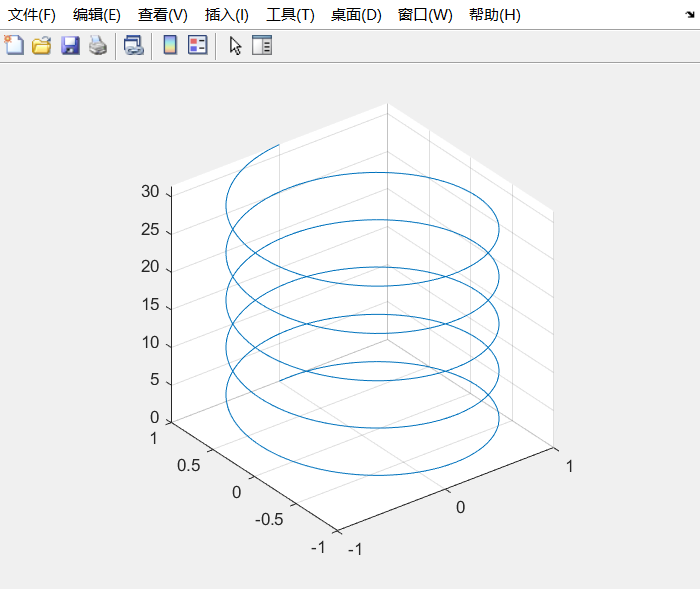示例2：螺旋图：
%% 螺旋状图(盘香)
clear; clc; close all;
turns = 40*pi;
t = linspace(0,turns,4000);
x = cos(t).*(turns-t)./turns;
y = sin(t).*(turns-t)./turns;
z = t./turns;
plot3(x,y,z); % 使用plot3绘制
grid on;

效果显示：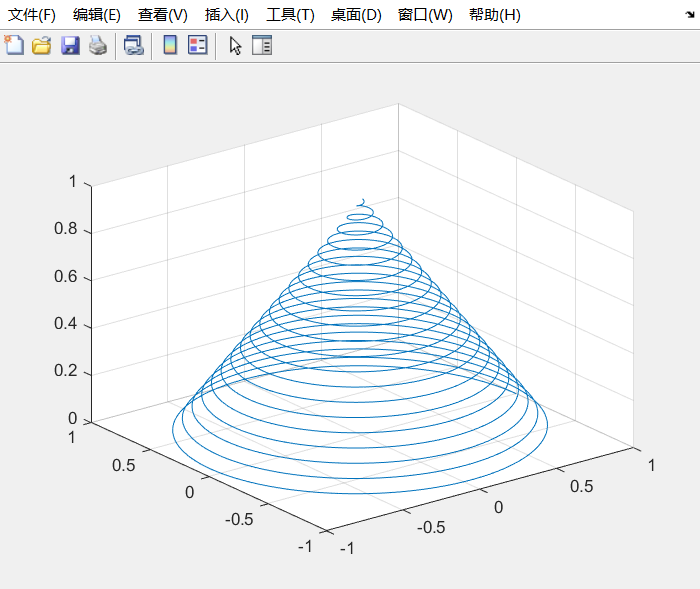2、通过meshgrid函数生成网格来比较surf函数和mesh函数的区别，了解meshgrid函数的用法
。示例：
%% 比较surf和mesh的区别
clear; clc; close all;
x = -3.5:0.2:3.5; y = -3.5:0.2:3.5;
[X,Y] = meshgrid(x,y);  % 获取网格
Z = X.*exp(-X.^2-Y.^2);  % 设定Z的范围
subplot(1,2,1);
mesh(X,Y,Z);  % 显示绘图的线，但是表格中间没有填充颜色
title('mesh draw');
subplot(1,2,2);
surf(X,Y,Z);  % 显示绘图的线，表格中间有填充的颜色
title('surf draw');

效果显示：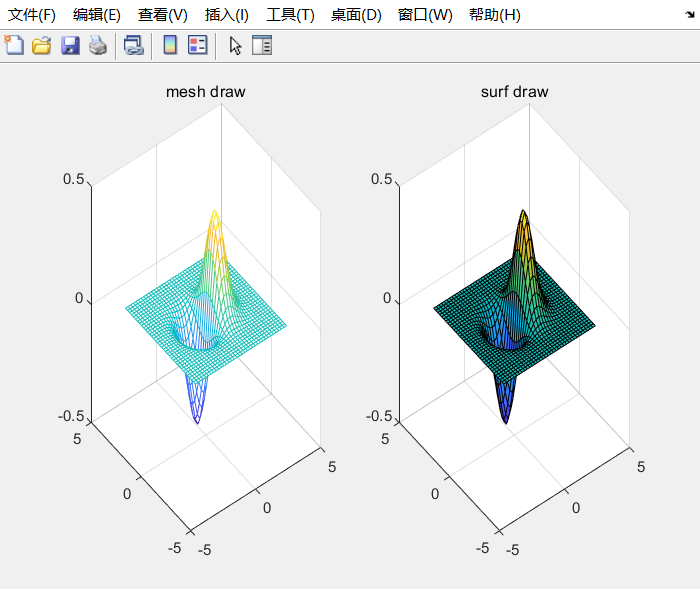想了解更多绘图信息，请看下面博文：
二维：
MATLAB二维绘图(一)使用plot函数进行简单绘图
MATLAB二维绘图(二)向图中添加标题，坐标轴，图标和文字信息
MATLAB二维绘图(三)操作绘图句柄显示特定的效果
MATLAB二维绘图(四)其他特殊的二维绘图函数介绍及用法
fill填充函数解析及用法示例
三维：
MATLAB三维绘图(二)使用隐函数作三维图
MATLAB三维绘图(三)绘制等值线图
MATLAB三维绘图(四)绘制特殊的三维图
MATLAB三维绘图(五)高级三维绘图


展开全文• MATLAB三维绘图(一)三维基础绘图三维图就是在维图的基础上多增加了一维，实质上在MATLAB中绘制的都是三维图。简单看一下维图是怎样变成三维图的，使用plot3函数可以很清楚的看出来。示例：%% 简单理解三维画图...
MATLAB三维绘图(一)三维基础绘图三维图就是在二维图的基础上多增加了一维，实质上在MATLAB中绘制的都是三维图。简单看一下二维图是怎样变成三维图的，使用plot3函数可以很清楚的看出来。示例：%% 简单理解三维画图clear; clc; close all;x=0:0.1:5*pi;z1=sin(x);z2=sin(2.*x);z3=sin(3.*x);y1=zeros(size(x));y3=ones(size(x));y2=y3./2;plot3(x,y1,z1,'r',x,y2,z2,'b',x,y3,z3,'g');  % 画出三条不同的直线显示的是三维图像grid on; % 显示网格xlabel('x-axis'); ylabel('y-axis'); zlabel('z-axis');效果显示：1、使用最简单的三维画图函数plot3来认识一下三维图。示例1：弹簧图%% 螺旋状图(弹簧)clear; clc; close all;t = 0:pi/50:10*pi;plot3(sin(t),cos(t),t);  % 使用plot3进行绘制三维曲线图grid on;   % 显示网格axis square;   % 坐标边框尺度大小相同效果显示：示例2：螺旋图：%% 螺旋状图(盘香)clear; clc; close all;turns = 40*pi;t = linspace(0,turns,4000);x = cos(t).*(turns-t)./turns;y = sin(t).*(turns-t)./turns;z = t./turns;plot3(x,y,z); % 使用plot3绘制grid on;效果显示：2、通过meshgrid函数生成网格来比较surf函数和mesh函数的区别，了解meshgrid函数的用法。示例：%% 比较surf和mesh的区别clear; clc; close all;x = -3.5:0.2:3.5; y = -3.5:0.2:3.5;[X,Y] = meshgrid(x,y);  % 获取网格Z = X.*exp(-X.^2-Y.^2);  % 设定Z的范围subplot(1,2,1);mesh(X,Y,Z);  % 显示绘图的线，但是表格中间没有填充颜色title('mesh draw');subplot(1,2,2);surf(X,Y,Z);  % 显示绘图的线，表格中间有填充的颜色title('surf draw');效果显示：展开全文• MATLAB三维绘图()使用隐函数作三维图 1、ezmesh函数：示例： clear; clc; close all; figure; ezmesh('x.*exp(x.^2-y.^2)'); 效果显示： 2、ezsurf函数：示例： clear; clc; close all; figure; ezsurf('x.*y.^3...
• 【数学建模】（四）：MATLAB二维绘图Tips矩阵及数组函数句柄plot绘图命令subplot(m,n,p)fplot()ezplot()不同坐标系极坐标双y坐标轴图形标注坐标轴控制设定范围填充 Tips 矩阵及数组 x=linspace(0,2*pi,100) %已知...数学建模 数据可视化
• ## MATLAB三维绘图

万次阅读 2018-08-29 16:05:54
绘制三维图像 一、plot3函数 x=0:pi/50:10*pi; sin=sin(x); cos=cos(x); plot3(sin,cos,x); title(‘helix’),text(0,0,0,’origin’); xlabel(‘sin(x)’),ylabel(‘cos(x)’),zlabel(‘x’) 、mesh...
• 目的：将matlab二维数组通过plot3形象的三维图表达 成果图如上图所示，黑色的线，红色的线和绿色的线分别代表数据集中的三个标签类别，横轴代表样本总数90个（图中没有截全，只能看到40左右），纵轴代表每个样本的...
• 文章目录一、前言、基本的绘图命令 plot1. 命令参数2. 示例演示3. 对坐标系进行调整、为图形加标注1. 参数说明2. 示例说明四、创建多幅子图五、其他图表 一、前言 通过 MATLAB 实现对下面图像的绘制： 、...数据可视化 plot...

# matlab二维绘图三维绘图matlab 订阅